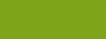﻿ Help 3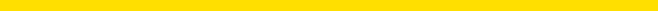Music Slide Rule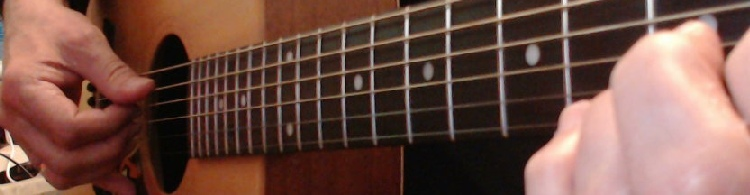HOME1
The Modes of the Major Scale can be found in an
easier way similar to the way the Pentatonic and
Blues Scales can be found

A-The Hard Way
By using the Major Scales and Modes Slide Rule
and plotting out the formulas shown for the
Ionian Mode*      =1. 2. 3. 4. 5. 6. 7. 1.
Dorian Mode       = 1. 2. b3. 4. 5. 6. b7. 1.
Phrygian Mode    =1. b2. b3. 4. 5. b6. b7. 1.
Lydian Mode       =1. 2. 3.  #4. 5. 6. 7. 1.
Mixolydian Mode =1. 2. 3. 4. 5.  6. b7. 1.
Aeolian Mode      =1. 2. b3. 4. 5. b6. b7.  1.
Locrian Mode      =1. b2. b3. 4. b5. b6. b7.  1.

*(Except for the Major Scale (also knkow as
The Ionian Mode)where you do use and

B-The Easy Way - Use the Major Scale Slide Rule

Remember with the Pentatonic scales I mentioned
that the 6th note of any Major Scale is known as the
relative minor e.g. in the Key of:
C Major. A minor is the relative minor.
(The relative minor is also known as the
Aeolian Mode (The 6th Mode))

So the Easy Way to find any Mode is:
To find the 1st Mode (The Major Scale. (Ionian Mode)
slide insert so the No. 1 is over the Key you want

To find the 2nd Mode  (The Dorian Mode)
slide insert so the No. 2 is over the Key you want

To find the 3rd Mode  (The Phrygian Mode)
slide insert so the No. 3 is over the Key you want

To find the 4th Mode  (The Lydian Mode)
slide insert so the No. 4 is over the Key you want

To find the 5th Mode  (The Mixoydian Mode)
slide insert so the No. 5 is over the Key you want

To find the 6th Mode  (The Aeolian Mode)
slide insert so the No. 6 is over the Key you want

To find the 7th Mode  (The Locrian Mode)
slide insert so the No. 7 is over the Key you want

See the Diagram 1 below.
Diagram 1  The Modes of the Major Scale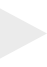HOME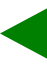2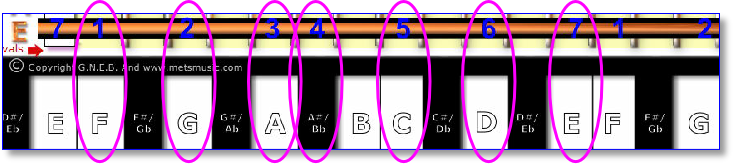3
Suggested reading for a more in depth
understanding of Practical Theory

1-F Major (Ionian)

2-G Dorian Mode

3-A Phrygian Mode

4-Bb Lydian Mode

5-C Mixolydian Mode

6-D Aeolian Mode

7-E Locrian Mode